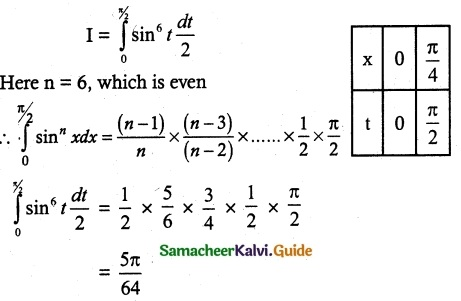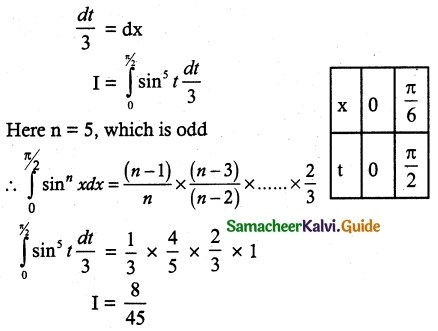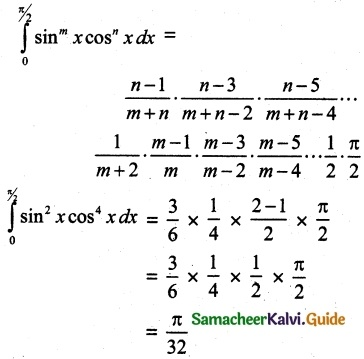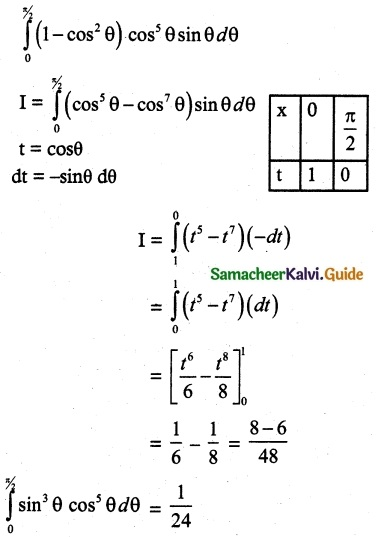Tamilnadu State Board New Syllabus Samacheer Kalvi 12th Maths Guide Pdf Chapter 9 Applications of Integration Ex 9.6 Textbook Questions and Answers, Notes.

## Tamilnadu Samacheer Kalvi 12th Maths Solutions Chapter 9 Applications of Integration Ex 9.6

Question 1.
Evaluate the following
(i) $$\int_{0}^{π/2}$$ sin10x dx
Solution:
Here n = 10, which is even(ii) $$\int_{0}^{π/2}$$ cos7x dx
Solution:
Here n = 7, which is odd(iii) $$\int_{0}^{π/4}$$ sin6 2x dx
Solution:
put t = 2x
dt = 2 dx(iv) $$\int_{0}^{π/6}$$ sin5 3x dx
Solution:
put t = 3x
dt = 3 dx(v) $$\int_{0}^{π/2}$$ sin2 x cos4 x dx
Solution:
Here m = 2, which is even and n = 4, which is even(vi) $$\int_{0}^{2π}$$ sin7 $$\frac{x}{4}$$ dx
Solution:(vii) $$\int_{0}^{π/2}$$ sin3 θ cos5 θ dθ
Solution:Aliter Method(viii) $$\int_{1}^{0}$$ x² (1 – x)³ dx
Solution:
By applying reduction formula